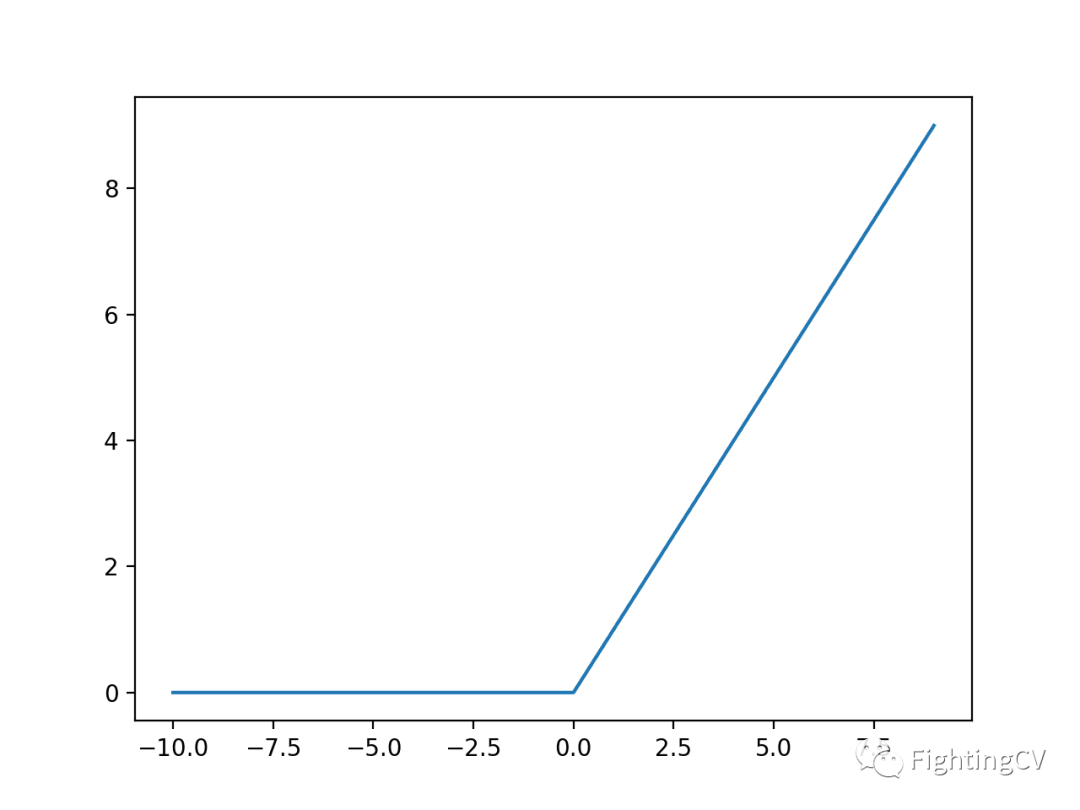1、Sigmoid 和 Tanh 激活函数的局限性

2、ReLU（Rectified Linear Activation Function）

3、如何实现ReLU

4、ReLU的优点

5、使用ReLU的技巧

# 1. Sigmoid 和 Tanh 激活函数的局限性

Sigmoid 激活函数 ，也被称为 Logistic函数神经网络，传统上是一个非常受欢迎的神经网络激活函数。函数的输入被转换成介于0.0和1.0之间的值。大于1.0的输入被转换为值1.0，同样，小于0.0的值被折断为0.0。所有可能的输入函数的形状都是从0到0.5到1.0的 s 形。在很长一段时间里，直到20世纪90年代早期，这是神经网络的默认激活方式。

Sigmoid和 tanh 函数的一个普遍问题是它们值域饱和了 。这意味着，大值突然变为1.0，小值突然变为 -1或0。此外，函数只对其输入中间点周围的变化非常敏感。

# 2. ReLU（Rectified Linear Activation Function）

``````if input > 0:
return input
else:
return 0
``````

# 3. 如何实现ReLU

``````# rectified linear function
def rectified(x):
return max(0.0, x)
``````

``````# demonstrate the rectified linear function

# rectified linear function
def rectified(x):
return max(0.0, x)

# demonstrate with a positive input
x = 1.0
print('rectified(%.1f) is %.1f' % (x, rectified(x)))
x = 1000.0
print('rectified(%.1f) is %.1f' % (x, rectified(x)))
# demonstrate with a zero input
x = 0.0
print('rectified(%.1f) is %.1f' % (x, rectified(x)))
# demonstrate with a negative input
x = -1.0
print('rectified(%.1f) is %.1f' % (x, rectified(x)))
x = -1000.0
print('rectified(%.1f) is %.1f' % (x, rectified(x)))
``````

``````rectified(1.0) is 1.0
rectified(1000.0) is 1000.0
rectified(0.0) is 0.0
rectified(-1.0) is 0.0
rectified(-1000.0) is 0.0
``````

``````# plot inputs and outputs
from matplotlib import pyplot

# rectified linear function
def rectified(x):
return max(0.0, x)

# define a series of inputs
series_in = [x for x in range(-10, 11)]
# calculate outputs for our inputs
series_out = [rectified(x) for x in series_in]
# line plot of raw inputs to rectified outputs
pyplot.plot(series_in, series_out)
pyplot.show()
``````ReLU函数的导数是斜率。负值的斜率为0.0，正值的斜率为1.0。

# 4. ReLU的优点

## 4.1. 计算简单性

tanh 和 sigmoid 激活函数需要使用指数计算， 而ReLU只需要max()，因此他计算上更简单，计算成本也更低 。

## 4.2. 代表性稀疏

ReLU的一个重要好处是，它能够输出一个真正的零值 。这与 tanh 和 sigmoid 激活函数不同，后者学习近似于零输出，例如一个非常接近于零的值，但不是真正的零值。这意味着负输入可以输出真零值，允许神经网络中的隐层激活包含一个或多个真零值。这就是所谓的稀疏表示，是一个理想的性质，在表示学习，因为它可以加速学习和简化模型。

## 4.3. 线性行为

ReLU看起来更像一个线性函数，一般来说，当神经网络的行为是线性或接近线性时，它更容易优化 。

## 4.4. 训练深度网络

ReLU的出现使得利用硬件的提升和使用反向传播成功训练具有非线性激活函数的深层多层网络成为可能 。

# 5. 使用ReLU的技巧

## 5.2. 对 MLPs，CNNs 使用 ReLU，但不是 RNNs

ReLU 可以用于大多数类型的神经网络，它通常作为多层感知机神经网络和卷积神经网络的激活函数 ，并且也得到了许多论文的证实。传统上，LSTMs 使用 tanh 激活函数来激活cell状态，使用 Sigmoid激活函数作为node输出。而ReLU通常不适合RNN类型网络的使用。

## 5.6. 使用惩罚权重

ReLU 的输出在正域上是无界的。这意味着在某些情况下，输出可以继续增长。因此，使用某种形式的权重正则化可能是一个比较好的方法，比如 l1或 l2向量范数。这对于提高模型的稀疏表示(例如使用 l 1正则化)和降低泛化误差都是一个很好的方法 。